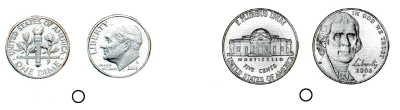# Texas Go Math Kindergarten Module 15 Assessment Answer Key

Refer to our Texas Go Math Kindergarten Answer Key Pdf to score good marks in the exams. Test yourself by practicing the problems from Texas Go Math Kindergarten Module 15 Assessment Answer Key.

## Texas Go Math Kindergarten Module 15 Assessment Answer Key

Concepts and Skills

Question 1.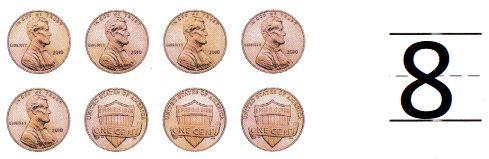Explanation:
1 penny is equal to 1 cent
There are 8 pennies.

Question 2.Explanation:
1 nickel is equal to 5 cents
there are 7 nickels.

Question 3.Explanation:
1 dime is equal to ten cents
there are 10 dimes.

Directions
1. Count the pennies. Write the number that shows how many pennies. TEKS K.4 2. Count the nickels. Write the number that shows how many nickels. TEKS K.4 3. Count the dimes. Write the number that shows how many dimes. TEKS K.4

Question 4.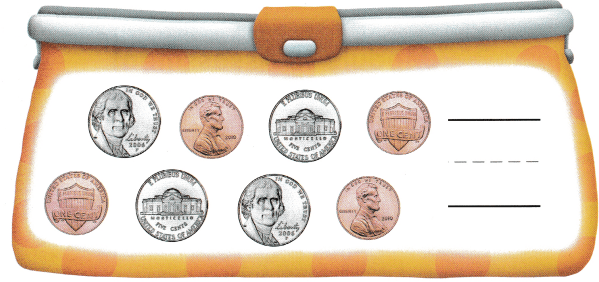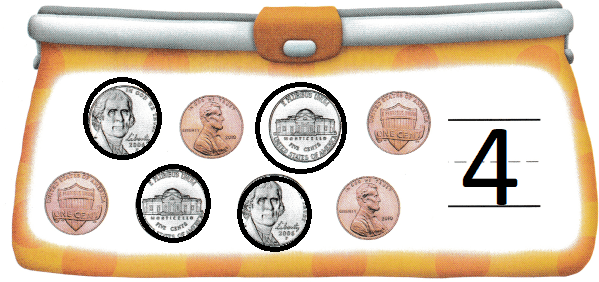Explanation:
In the pouch there are nickels and pennies
so, circled the pennies
There are 4 pennies.

Question 5.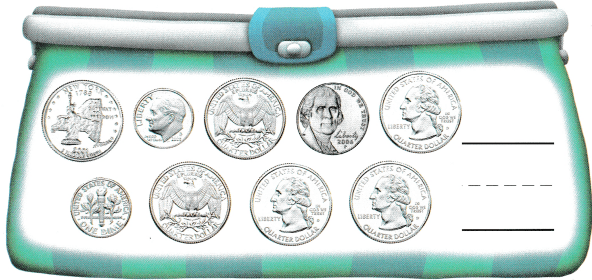Explanation:
1 quarter dollar = 25 cents
In the bag there are quarter coins and nickels
so, circled the quarter coins
there are 6 quarter dollars

Question 6.
Texas test Prep